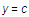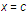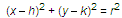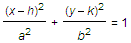# EquationSelection prerequisites: One or more lines or circles

This Measure menu command measures the equation of each selected object with respect to the marked coordinate system.

If there is no marked coordinate system, Sketchpad marks an existing coordinate system or creates a new one.

The equation of a line is expressed in one of the following three forms:for a horizontal linefor a vertical linefor any other line

For a circle, the equation is expressed in one of the following two forms:In other words, a Euclidean circle described by an equation in square coordinates has a circle’s equation. The same shape, described in nonsquare coordinates, has the equation of an ellipse.